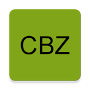# Code Breaker Z APK

1.0.2 for Android 4.1 and up

## This is a code breaking game like Mastermind, CodeBreaker, Bulls and Cows, etc.

Package name: jp.gr.java_conf.hysktht.mycodebreaker2

This is a code breaking game.
Let's continue your winning streak !

Code Breaker Z (CBZ) is a game that guess the hidden numbers.
This type of game was popular for a long time as the name of Mastermind, Hit and Blow, Bulls and Cows, Code Breaker, Numeron, AB, Namdy, and so on.

Enter three different numbers and press "CALL". If the place(order) and number are correct, it is H. Or If the number is correct but if the place is different, it is B. the count of H and B is displayed. Based on the displayed hints, predict the correct answer and enter it again.
It is successful if you answer within 6 times!

・ Battle with yourself
・ No time limit
・ Easy to use simple screen
・ Please think through logic and probability theory

You can get a star by extending winning streak.
And new options (level) will be available.

※ To drop the game is "FOLD".
It may be regarded as a failure if you terminate the application in the middle.
Changing the level will result in no winning streak.
"FOLD" decreases FP (Fold Point).
FP increases over time or by watching advertisements.

Let's complete by extending the winning streak record!
(30 wins at all levels)

Below is an explanation of how to play for beginners.

Example: Numbers 1 to 4 are used.
[1, 2, 3] => [0 H 2 B]
[2, 3, 4] => [0 H 2 B]
When this is the case, how can we guess the next?

Looking at the first hint, you can see that two of 1, 2, 3 are used. Conversely, since only two are used, the remaining one is 4.
Looking at the second hint, you can see that two of 2, 3, 4 are used. Conversely, since only two are used, the remaining one is 1.

It turned out that 4 and 1 are used so far. Regardless of the location, the three numbers in use are either "4, 1, 2" or "4, 1, 3".

Next, think about the place.
Let's look at the pattern of "4, 1, 2". Where is the place of 2? If it is left it should be H for the second hint. If it's in the middle, it should be H for the first hint. Therefore 2 is the only place to put in the right.
Where is 1 place? If it is left, the first hint should be H. The right has been decided to 2. Therefore 1 is the only place to put in the middle.

That means that only "4, 1, 2" will be candidates for answers.

However, patterns of "4, 1, 3" are also possible. Please think in the same way. Then, the left is only place to put 3. That means that there is only place of 4 in the middle. As a result, only "3, 4, 1" is a candidate for answers.

From the above it is understood that either "4, 1, 2", "3, 4, 1" is the correct answer.
When it gets here, the rest is intuition. Let's enter the Answer what thinks it is correct.
What's New
ver 1.0.2 FP(Fold Point) & AD

Category:

Date published: Jan 14, 2019

Current version: 1.0.2

Requires android: 4.1

Content rating: Everyone

4.6
3 total
5 2
4 1
3 0
2 0
1 0